# What is the difference between Parse Tree and the Syntax Tree?

Parse Tree

Parse tree is a hierarchical structure that defines the derivation of the grammar to yield input strings. In parsing, the string is derived using the start symbol. The root of the parse tree is that start symbol. It is the graphical description of symbols that can be terminals or non-terminals. Parse tree follows the precedence of operators. The deepest sub-tree traversed first. Therefore, the operator in the parent node has less precedence over the operator in the sub-tree.

A Parse Tree for a CFG G = (V, Σ, P, S) is a tree satisfying the following conditions −

• Root has the label S, where S is the start symbol.
• Each vertex of the parse tree has a label which can be a variable (V), terminal (Σ) or ε.
• If A → C1, C2 … … . Cn is a production, then C1, C2 … … . Cn are children of node labeled A.
• Leaf Nodes are terminal (Σ), and Interior nodes are variable (V).
• The label of an internal vertex is always a variable.
• If a vertex A has k children with labels A1, A2 … … . Ak, then A → A1, A2 … … . Ak will be production in context-free grammar G.

Syntax Tree

A syntax tree is a tree that displays the syntactic structure of a program while ignoring inappropriate analysis present in a parse tree. Thus, the syntax tree is nothing more than a condensed form of the parse tree. The operator and keyword nodes of a parse tree are shifted to their parent and a group of individual production is replaced by an individual link. For example, for a given parse tree of string id + id * id.

Syntax Tree for the expression is as follows −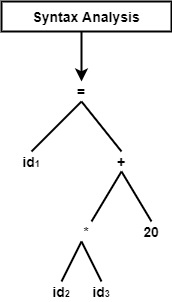Example− Construct

• Parse Tree

• Syntax Tree

• Annotated for complete parse tree for the input string 1 * 2 + 3 by using any grammar you know.

## Solution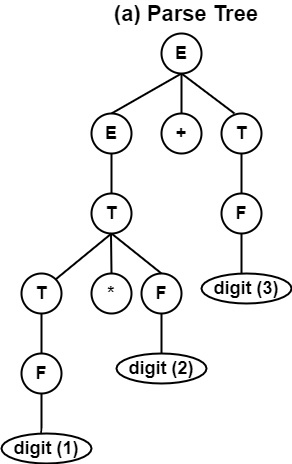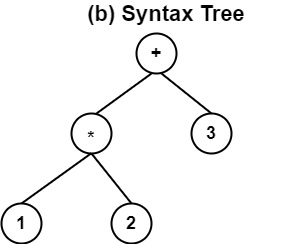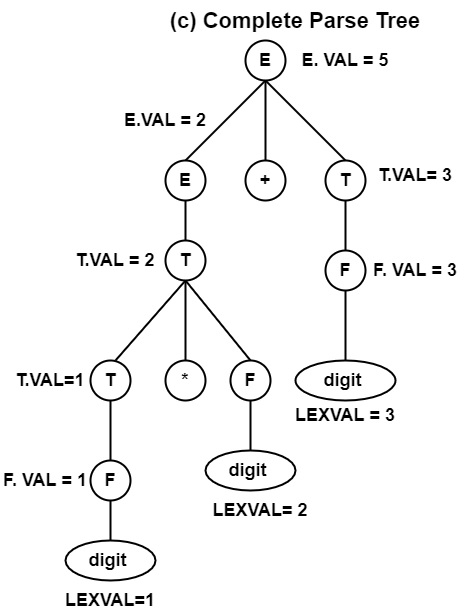Let us see the comparison between the Parse tree and Syntax Tree.

Parse TreeSyntax Tree
It can contain operators & operands at any node of the tree, i.e., either interior node or leaf node.It contains operands at leaf node & operators as interior nodes of Tree.
It contains duplicate or redundant information.It does not include any redundant data.
Parse Tree can be changed to Syntax Tree by the elimination of redundancy, i.e., by compaction.Syntax Tree cannot be changed to Parse Tree.
Example− 1 *2 + 3.Example− 1 *2 + 3.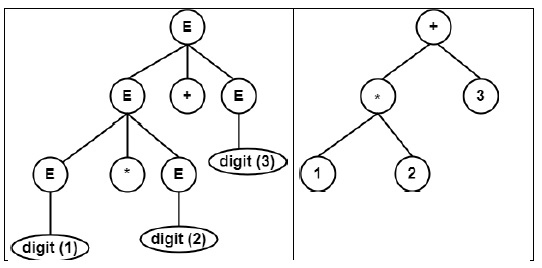Updated on: 05-Nov-2021

14K+ Views